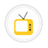you are here->home->Physical Sciences->Electric Circuits Virtual Lab (Pilot)
Electric Circuits Virtual Lab (Pilot)

An electric circuit is composed of individual electrical components such as resistors, inductors, capacitors etc to trace the current that flows through it. The combination of electrical components can perform various simple and compound electrical operations.Parallel RC CircuitsThe parallel RC circuit is generally of less interest than the series circuit. This is largely because the output voltage Voutis equal to the input voltage Vin as a result, this circuit does not act as a filter on the input signal unless fed by a currentParallel LC CircuitsAn LC circuit is a resonant circuit or tuned circuit that can store electrical energy vibrating at its resonant frequency. In parallel LC circuit, coil (L) and capacitor (C) are connected in parallel with an AC power supply. A parallel resonant circuit prThevenin’s TheoremIn electrical circuit theory, Thévenin's theorem for linear electrical networks states that any combination of voltage sources, current sources and resistors with two terminals is electrically equivalent to a single voltage source V and a single serSeries RL CircuitsA Resistor-Inductor (RLcircuit) or RL filter or RL network, is one of the simplest analogue infinite impulse response electronic filters.A series resistance R and self-inductance L is driven by an input voltage.Norton's theoremNorton's theorem for electrical networks states that any collection of voltage sources, current sources, and resistors with two terminals is electrically equivalent to an ideal current source, I, in parallel with a single resistor, R. For single-freqSeries LCR CircuitsSeries LCR CircuitsKirchhoff’s LawsKirchhoff's circuit laws are two equalities that deal with the conservation of charge and energy in electrical circuits, and were first described in 1845 by Gustav Kirchhoff.Series RC CircuitsThese equations show that a series RC circuit has a time constant, usually denoted τ = RC being the time it takes the voltage across the component to either rise (across C) or fall (across R) to within 1 / e of its final value.Series LC CircuitsHere R, L, and C are in series in an ac circuit. Inductive reactance magnitude () increases as frequency increases while capacitive reactance magnitude () decreases with the increase in frequency. A series resonant circuit provides voltage magnification.Parallel LCR CircuitsA parallel RLC circuit is an example of a band-stop circuit response that can be used as a filter to block frequencies at the resonance frequency but allow others to pass.Parallel RL CircuitsThe parallel RL circuit is generally of less interest than the series circuit unless fed by a current source. This is largely because the output voltage Vout is equal to the input voltage Vin— as a result, this circuit does not act as a filter for a volCopyright @ 2019 Under the NME ICT initiative of MHRDPowered by AmritaVirtual Lab Collaborative Platform [ Ver 00.13. ]